# AP Physics C: Mechanics : Momentum and Impulse

## Example Questions

### Example Question #1 : Momentum And Impulse

A baseball player hits a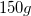baseball initially moving at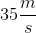, returning it at a speed of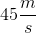along the same path. If the ball was in contact with the bat for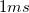, what magnitude of force did the ball experience during the moment of contact?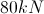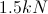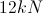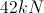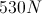Explanation:

Relevant equations: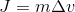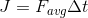Evaluate the impulse based on the mass and change of velocity.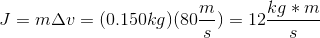Use the total impulse and time in the second equation.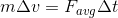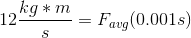Solve for the average force.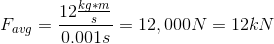### Example Question #1 : Momentum And Impulse

Which of the following could be used as units of impulse?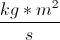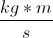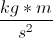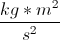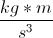Explanation:

Relevant equations: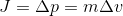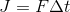Impulse is defined as change in momentum, so has the same units as momentum. These units can easily be found using the given equations.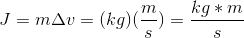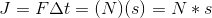### Example Question #3 : Momentum And Impulse

A pitcher throws a 0.15kg baseball at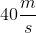towards the batter and the batter hits the ball with his bat. The ball leaves the bat in the opposite direction at a speed of. Calculate the impulse experienced by the baseball.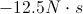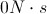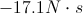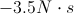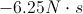Explanation:

To calculate the impulse, know that it is equal to the change in momentum.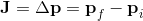Write the impulse equation in terms of mass and velocity.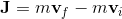In our case, the initial velocity of the baseball is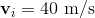and its final velocity is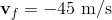, where the negative sign indicates that the ball is traveling in the opposite direction. Also, m = 0.15kg. The impulse on the ball can be calculated below.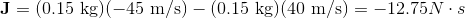The negative sign tells that the force on the baseball is opposed to the original momentum.